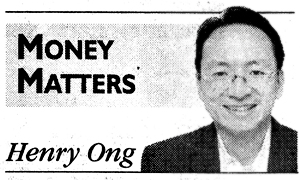# How to Profit with Benjamin Graham FormulaThere are many ways to determine the intrinsic value of a stock. The process of finding the “true” worth of a company, however, may mean different things to different types of investors.

In my column last week titled “Deep Value Investing,” I mentioned that the legendary value investor. Benjamin Graham, believed that investing in stocks that trade less than their intrinsic values could yield potential returns in the long run.

Graham came up with different approaches in calculating the intrinsic value of a stock. One of the methods he used, aside from the “net-net strategy” that we discussed last week, was the earnings multiplier.

This multiplier, now known as the Benjamin Graham formula, estimates the intrinsic value of a stock by multiplying the current earnings of a company with the factor (8.5 + 2g).

Graham assumed that the P/E ratio of a no-growth stock was 8.5. He then incorporated growth into the multiplier by adding two times the minimum future growth rate of a company.

For example, if we want to estimate the intrinsic value of Megaworld, we simply multiply the company’s sustainable growth rate of 8.2 percent by 2 to get absolute value of 16.4. This is then added to 8.5 to derive an earnings multiplier of 24.9.

Using this multiplier against trailing earnings per share of Megaworld at P0.44, we can derive the intrinsic value of the stock at P10.9, which offers a huge 56 percent discount to its current share price.

Graham used this formula for some time until he updated and revised this after more than 10 years by including the interest rate factor.

Based on his revision, the estimated value from the original formula should be modified by the ratio of 4.4 and the current 10-year bond yield, which should look like this: (8.5+2g) x 4.4/yield.

Following this new formula with the current 10-year bond yield at 7.07 percent, the interest rate factor shall be 0.62, which is derived by simply dividing 4.4 with 7.07.

Using the interest rate factor against the earlier computed value of Megaworld at P10.9, we now have a revised intrinsic value of P6.80, which still offers a big 29 percent discount to its current share price.

Remember that this formula was developed more than 50 years ago and assumptions used then may no longer be applicable to the present.

So how reliable is the Graham formula in today’s market?

If we computed the margin of safety of the 30 PSE Index stocks by end of 2016 using the Graham formula, we would know that the market at that time was undervalued by median of 6 percent.

Because most stocks were trading below their supposed intrinsic values, you would expect share prices to recover in the near term, which the market did with a median return of 24 percent after one year.

In the following year, by end of 2017, we again applied the same formula. This time, the corresponding historical results would indicate that the market was overvalued by 17 percent premium.

Knowing the market to be expensive by Graham’s standards, you would expect share prices to correct soon. True enough, the PSE index fell this year by as much as 20 percent.

How about the prospects of stock market next year?

Based on Graham’s valuation, the market, having recovered from its historical lows recently, is slightly overvalued at current prices with median premium of 8 percent.

While the year is not yet over, valuation may still improve as market expects interest rates to decline further.

As the yield on 10-year bonds decreases, the earnings multiplier increases. A higher multiplier means higher margin of safety, which makes the market cheaper.

Perhaps the current correction may be an opportunity to buy?

Though the Graham formula may still seem to be relevant to this day, this is not a perfect tool in calculating intrinsic values.

Understanding the concepts behind the formula is more useful because it can guide you in making investment decisions about the future.

As Benjamin Graham said, “In the short run, the market is a voting machine but in the long run, it is a weighing machine.”Henry Ong is a Registered Financial Planner of RFP Philippines. He is one of best selling book co-author of Money Matters. He also writes regularly as columnist for the Philippine Daily Inquirer.

747 total views, 2 views today